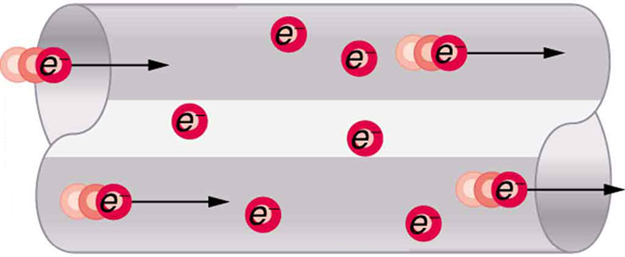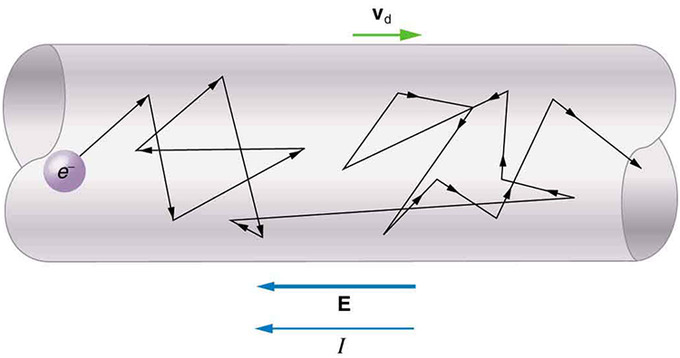# Electric Current

## The Battery

A battery is a device that converts chemical energy directly to electrical energy.

### Learning Objectives

Describe the functions and identify the major components of a battery

### Key Takeaways

#### Key Points

• A battery stores electrical potential from the chemical reaction. When it is connected to a circuit, that electric potential is converted to kinetic energy as the electrons travel through the circuit.
• The voltage or potential difference between two points is defined to be the change in potential energy of a charge q moved from point 1 to point 2, divided by the charge.
• The voltage of a battery is synonymous with its electromotive force, or emf. This force is responsible for the flow of charge through the circuit, known as the electric current.

#### Key Terms

• battery: A device that produces electricity by a chemical reaction between two substances.
• current: The time rate of flow of electric charge.
• voltage: The amount of electrostatic potential between two points in space.Symbol of a Battery in a Circuit Diagram: This is the symbol for a battery in a circuit diagram. It originated as a schematic drawing of the earliest type of battery, a voltaic pile. Notice the positive cathode and negative anode. This orientation is important when drawing circuit diagrams to depict the correct flow of electrons.

A battery is a device that converts chemical energy directly to electrical energy. It consists of a number of voltaic cells connected in series by a conductive electrolyte containing anions and cations. One half-cell includes electrolyte and the anode, or negative electrode; the other half-cell includes electrolyte and the cathode, or positive electrode. In the redox (reduction-oxidation) reaction that powers the battery, cations are reduced (electrons are added) at the cathode, while anions are oxidized (electrons are removed) at the anode. The electrodes do not touch each other but are electrically connected by the electrolyte. Some cells use two half-cells with different electrolytes. A separator between half-cells allows ions to flow, but prevents mixing of the electrolytes.

Each half-cell has an electromotive force (or emf), determined by its ability to drive electric current from the interior to the exterior of the cell. The net emf of the cell is the difference between the emfs of its half-cells, or the difference between the reduction potentials of the half-reactions.

The electrical driving force across the terminals of a cell is known as the terminal voltage (difference) and is measured in volts. When a battery is connected to a circuit, the electrons from the anode travel through the circuit toward the cathode in a direct circuit. The voltage of a battery is synonymous with its electromotive force, or emf. This force is responsible for the flow of charge through the circuit, known as the electric current.

A battery stores electrical potential from the chemical reaction. When it is connected to a circuit, that electric potential is converted to kinetic energy as the electrons travel through the circuit. Electric potential is defined as the potential energy per unit charge (q). The voltage, or potential difference, between two points is defined to be the change in potential energy of a charge q moved from point 1 to point 2, divided by the charge. Rearranged, this mathematical relationship can be described as:

$\Delta \text{PE} =\text{q}\Delta \text{V}$

Voltage is not the same as energy. Voltage is the energy per unit charge. Thus a motorcycle battery and a car battery can both have the same voltage (more precisely, the same potential difference between battery terminals), yet one stores much more energy than the other. The car battery can move more charge than the motorcycle battery, although both are 12V batteries.

Ideal and Real Batteries: A brief introduction to ideal and real batteries for students studying circuits.

## Current and Voltage Measurements in Circuits

The electrical current is directly proportional to the voltage applied and inversely related to the resistance in a circuit.

### Learning Objectives

Describe the relationship between the electrical current, voltage, and resistance in a circuit

### Key Takeaways

#### Key Points

• A simple circuit consists of a voltage source and a resistor.
• Ohm ‘s law gives the relationship between current I, voltage V, and resistance R in a simple circuit: I = V/R.
• The SI unit for measuring the rate of flow of electric charge is the ampere, which is equal to a charge flowing through some surface at the rate of one coulomb per second.

#### Key Terms

• electrical current: the movement of charge through a circuit
• ohm: in the International System of Units, the derived unit of electrical resistance; the electrical resistance of a device across which a potential difference of one volt causes a current of one ampere; symbol: Ω
• ampere: A unit of electrical current; the standard base unit in the International System of Units. Abbreviation: amp. Symbol: A.

To understand how to measure current and voltage in a circuit, you must also have a general understanding of how a circuit works and how its electrical measurements are related.

What is Voltage?: This video helps with a conceptual understanding of voltage.

An electrical circuit is a type of network that has a closed loop, which provides a return path for the current. A simple circuit consists of a voltage source and a resistor and can be schematically represented as in.A Simple Circuit: A simple electric circuit made up of a voltage source and a resistor

According to Ohm’s law, The electrical current I, or movement of charge, that flows through most substances is directly proportional to the voltage V applied to it. The electric property that impedes current (crudely similar to friction and air resistance) is called resistance R. Collisions of moving charges with atoms and molecules in a substance transfer energy to the substance and limit current. Resistance is inversely proportional to current. Ohm’s law can therefore be written as follows:

$\text{I} = \text{V}/\text{R}$

where I is the current through the conductor in amperes, V is the potential difference measured across the conductor in volts, and R is the resistance of the conductor in ohms (Ω). More specifically, Ohm’s law states that R in this relation is constant, independent of the current. Using this equation, we can calculate the current, voltage, or resistance in a given circuit.

For example, if we had a 1.5V battery that was connected in a closed circuit to a lightbulb with a resistance of 5Ω, what is the current flowing through the circuit? To solve this problem, we would just substitute the given values into Ohm’s law: I = 1.5V/5Ω; I = 0.3 amperes. If we know the current and the resistance, we can rearrange the Ohm’s law equation and solve for voltage V:

$\text{V} = \text{IR}$

## A Microscopic View: Drift Speed

The drift velocity is the average velocity that a particle achieves due to an electric field.

### Learning Objectives

Relate the drift velocity with the velocity of free charges in conductors

### Key Takeaways

#### Key Points

• There is an electric field in conductors that causes electrons to drift in the direction opposite to the field. The drift velocity is the average velocity of these free charges.
• The expression for the relationship between the current and drift velocity can be obtained by considering the number of free charges in a segment of wire.
• I = qnAv relates the drift velocity to the current, where I is the current through a wire of cross-sectional area A made of a material with a free charge density n. The carriers of the current each have a charge q and move with a drift velocity of magnitude v.

#### Key Terms

• drift velocity: The average velocity of the free charges in a conductor.

### Drift Speed

Electrical signals are known to move very rapidly. Telephone conversations carried by currents in wires cover large distances without noticeable delays. Lights come on as soon as a switch is flicked. Most electrical signals carried by currents travel at speeds on the order of 108m/s, a significant fraction of the speed of light. Interestingly, the individual charges that make up the current move much more slowly on average, typically drifting at speeds on the order of 10−4m/s.

The high speed of electrical signals results from the fact that the force between charges acts rapidly at a distance. Thus, when a free charge is forced into a wire, the incoming charge pushes other charges ahead of it, which in turn push on charges farther down the line. The resulting electrical shock wave moves through the system at nearly the speed of light. To be precise, this rapidly moving signal or shock wave is a rapidly propagating change in the electric field.Electrons Moving Through a Conductor: When charged particles are forced into this volume of a conductor, an equal number are quickly forced to leave. The repulsion between like charges makes it difficult to increase the number of charges in a volume. Thus, as one charge enters, another leaves almost immediately, carrying the signal rapidly forward.

### Drift Velocity

Good conductors have large numbers of free charges in them. In metals, the free charges are free electrons. The distance that an individual electron can move between collisions with atoms or other electrons is quite small. The electron paths thus appear nearly random, like the motion of atoms in a gas. However, there is an electric field in the conductor that causes the electrons to drift in the direction shown (opposite to the field, since they are negative). The drift velocity vdis the average velocity of the free charges after applying the field. The drift velocity is quite small, since there are so many free charges. Given an estimate of the density of free electrons in a conductor (the number of electrons per unit volume), it is possible to calculate the drift velocity for a given current. The larger the density, the lower the velocity required for a given current.Drift Speed: Free electrons moving in a conductor make many collisions with other electrons and atoms. The path of one electron is shown. The average velocity of the free charges is called the drift velocity and is in the direction opposite to the electric field for electrons. The collisions normally transfer energy to the conductor, requiring a constant supply of energy to maintain a steady current.

It is possible to obtain an expression for the relationship between the current and drift velocity by considering the number of free charges in a segment of wire. The number of free charges per unit volume is given the symbol n and depends on the material. Ax is the volume of a segment, so that the number of free charges in it is nAx. The charge ΔQ in this segment is thus qnAx, where q is the amount of charge on each carrier. (Recall that for electrons, q is 1.60×10−19C. ) The current is the charge moved per unit time. Thus, if all the original charges move out of this segment in time t, the current is:

$\text{I} = \Delta \text{Q}/\Delta \text{t} = \text{qnAx}/\Delta \text{t}$

Notably, x/Δt is the magnitude of the drift velocity vd, since the charges move an average distance x in a time t. Rearranging terms gives: I = qnAvd, where I is the current through a wire of cross-sectional area A made of a material with a free charge density n. The carriers of the current each have charges q and move with a drift velocity of magnitude vd.

Current density is the electric current per unit area of cross-section. It has units of Amperes per square meter.